VBA Randomize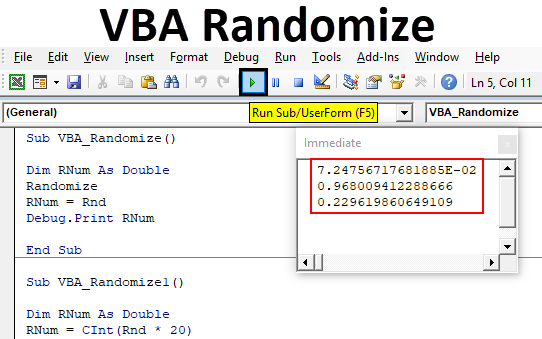Excel VBA Randomize

VBA Randomize is the function which is used for creating random number function which is RND. We all know that VBA RND is used for creating the random numbers which are mainly used for Billing, raising ticket numbers and many for applications are there. Here, VBA Randomize is used for changing the seed value which we feed for RND function. For better understanding, we are using RND function to get the random numbers then using Randomize function with RND which will give more variety of random numbers. As it changes in the seed input value by which the output also get changed. Which means, it will generate the numbers as per the seed input is given in RND function and the number generated will be in the same range.

The main thing about VBA Randomize is that it does not have any syntax. We just need to use it along with RND function which has the syntax to apply.

How to Randomize a Number in Excel VBA?

Below are the different examples to Randomize a number in excel using VBA Rnd Function.

You can download this VBA Randomize Excel Template here – VBA Randomize Excel Template

Excel VBA Randomize – Example #1

It is very easy to create customize random numbers by VBA Randomize. For this, follow the below steps:

Step 1: Go to the VBA window, under the Insert menu tab select Module as shown below.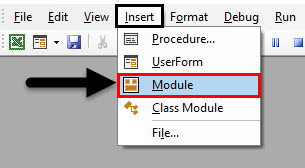Step 2: Now write the subcategory of VBA Randomize or we can use any name to define the VBA code.

Code:

Sub VBA_Randomize()

End Sub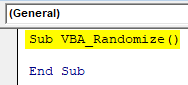Step 3: Now define a variable. Here, we have considered the variable RNum as data type Double. The logic behind using Double is that we will get minor changes in generated random numbers. Double will help us to see the numbers in decimals.

Code:

Sub VBA_Randomize()

Dim RNum As Double

End Sub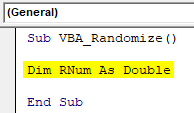Step 4: Now assign the VBA RND function to defined variable RNum.

Code:

Sub VBA_Randomize()

Dim RNum As Double
RNum = Rnd

End Sub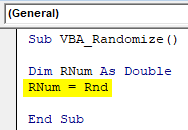Step 5: Now to see the generation of random numbers, we will use Debug.Print which is used for printing the message.

Code:

Sub VBA_Randomize()

Dim RNum As Double
RNum = Rnd
Debug.Print RNum

End Sub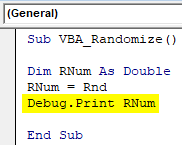Step 6: To see the value or number generation, open the Immediate Window from the View menu list. Or we can use a short cut key as Ctrl + G to get this window.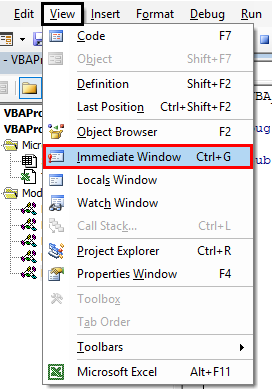Step 7: Now compile the code and run it by clicking on the Play button located below the menu bar. We will see the first random number in immediate window as shown below.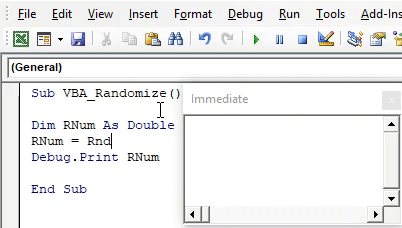And if we run the code, again and again, multiple times, then we would see a few more random numbers.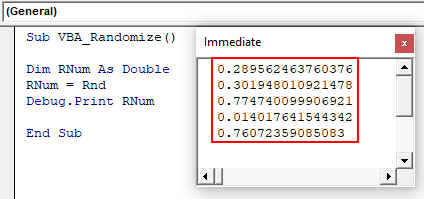Step 8: Now if we apply Randomize before RND function, then it will change the seed input which RND function was getting.

Code:

Sub VBA_Randomize()

Dim RNum As Double
Randomize
RNum = Rnd
Debug.Print RNum

End Sub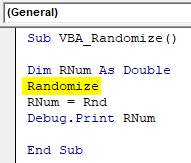Step 9: Again run the code multiple times to see what numbers are getting generated.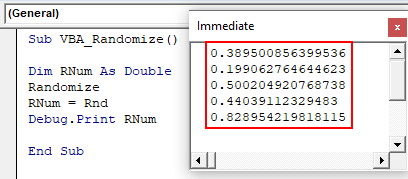This is how Randomize function works in VBA if used with RND function.

Let’s see some more experiment with the same coding. We will now use CInt function with RND which is used for Data type conversion. Which means, it will convert the generated random number as Double into Integers.

Code:

Sub VBA_Randomize1()

Dim RNum As Double
RNum = CInt(Rnd)
Debug.Print RNum

End Sub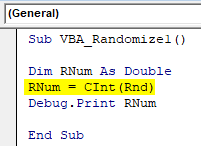Now again run the code. We will see, now the random numbers are getting generated as Integers. But the values are in the range of 0 and 1.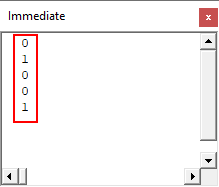We have already seen, if we keep on using the Double, then the values were coming in between 0 to 1. This is because we used Randomize along with RND function. Now let’s multiply the RND function with any number. Let’s say 20.

Code:

Sub VBA_Randomize1()

Dim RNum As Double
RNum = CInt(Rnd * 20)
Debug.Print RNum

End Sub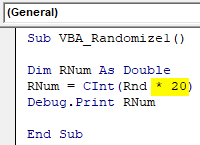Now again run the code.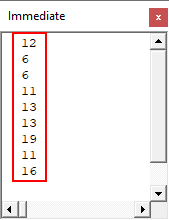Now the scene is changed. The random values generated are greater than 0 but are less than 20.

Excel VBA Randomize – Example #2

There is another way to see how VBA Randomize works. We will apply some mathematical formula along with Randomize and see how to randomize helps in generating random values. But in this example, we will see the output in the message box. For this, follow the below steps:

Step 1: In a module, write the subcategory of VBA Randomize as shown below.

Code:

Sub VBA_Randomize2()

End Sub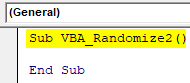Step 2: Consider the same variable which we defined in the last example but as Integer.

Code:

Sub VBA_Randomize2()

Dim RNum As Integer

End Sub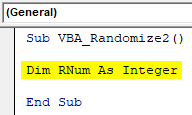Step 3: Now select the Randomize function here before we start putting mathematical formula.

Code:

Sub VBA_Randomize2()

Dim RNum As Integer
Randomize

End Sub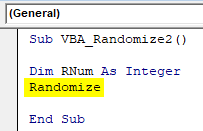Step 4: Now consider any mathematical formula such as addition, subtraction as per your requirement as shown below.

Code:

Sub VBA_Randomize2()

Dim RNum As Integer
Randomize
RNum = Int((300 - 200 + 1)

End Sub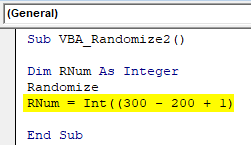Step 5: Now use RND function as shown below.

Code:

Sub VBA_Randomize2()

Dim RNum As Integer
Randomize
RNum = Int((300 - 200 + 1) * Rnd + 200)

End Sub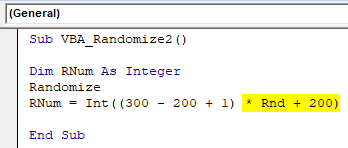You have noticed that we have used most of the mathematical expression which is generally used.

Step 6: Now use Msgbox with a defined variable to see the generated Random numbers.

Code:

Sub VBA_Randomize2()

Dim RNum As Integer
Randomize
RNum = Int((300 - 200 + 1) * Rnd + 200)
MsgBox RNum

End Sub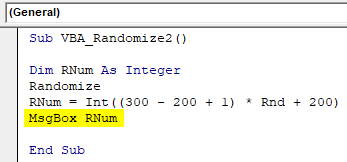Step 7: Now run the code. We will get a random number as 234. This is because the number is multiplied by (300-200+1) and then added with 200. Which means that the random number is quite less in nature and because of mathematical expressions used, it is coming as 234.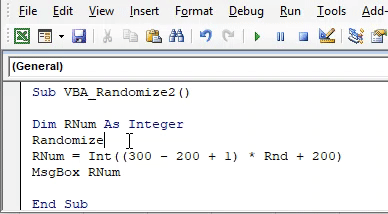And we run the code again, it will give us the message as 294.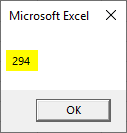Pros of Excel VBA Randomize

• We can generate any random number between any ranges we want.
• As the range becomes limited but still there is no limit on the generation of random numbers.
• We can limit the range of random number generation which would be greater than 0.

Things to Remember

• Randomize can be used with different functions as well. But, using this with RND function gives the result which we need.
• Randomize gives random numbers between 0 and 1 if used alone with RND.
• If we use any other number or mathematical expression with RND, then Randomize will be generated the random numbers between the highest value could be generated.
• Once done, save the file in Macro Enable excel format.
• Randomize can be used where need to generate the random numbers but between some range seed inputs.

Recommended Articles

This is a guide to VBA Randomize. Here we discuss how to Randomize a number in Excel using VBA code along with practical examples and downloadable excel template. You can also go through our other suggested articles –

1. Write Text File in Excel VBA
2. Generate Random Numbers in Excel
3. Excel VBA Variable Declaration
4. Excel Leading Zeros (Examples)

The post VBA Randomize appeared first on EDUCBA.

This post first appeared on Free Online CFA Calculator Training Course | EduCB, please read the originial post: here

Subscribe to Free Online Cfa Calculator Training Course | Educb

Get updates delivered right to your inbox!

×